### 1 决策树的基本思想

#### 1.1 冠军球队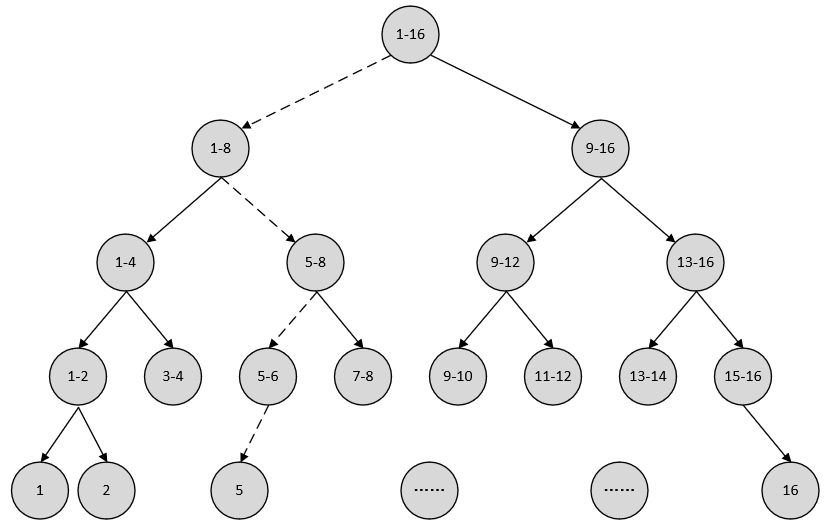#### 1.2 信息的度量

1) 信息熵

$H=-\left({p}_{1}\cdot \mathrm{log}{p}_{1}+{p}_{2}\cdot \mathrm{log}{p}_{2}+\cdots +{p}_{16}\cdot \mathrm{log}{p}_{16}\right)\phantom{\rule{0.278em}{0ex}}\phantom{\rule{0.278em}{0ex}}\phantom{\rule{0.278em}{0ex}}\phantom{\rule{0.278em}{0ex}}\phantom{\rule{0.278em}{0ex}}\phantom{\rule{0.278em}{0ex}}\phantom{\rule{0.278em}{0ex}}\left(8.1\right)$

$H\left(X\right)=-\sum _{x\in X}P\left(x\right)\mathrm{log}P\left(x\right)\phantom{\rule{0.278em}{0ex}}\phantom{\rule{0.278em}{0ex}}\phantom{\rule{0.278em}{0ex}}\phantom{\rule{0.278em}{0ex}}\phantom{\rule{0.278em}{0ex}}\phantom{\rule{0.278em}{0ex}}\phantom{\rule{0.278em}{0ex}}\phantom{\rule{0.278em}{0ex}}\phantom{\rule{0.278em}{0ex}}\left(8.2\right)$

$H\left(y\right)=-\left(p\mathrm{log}p+\left(1-p\right)\mathrm{log}\left(1-p\right)\right)\phantom{\rule{0.278em}{0ex}}\phantom{\rule{0.278em}{0ex}}\phantom{\rule{0.278em}{0ex}}\phantom{\rule{0.278em}{0ex}}\phantom{\rule{0.278em}{0ex}}\phantom{\rule{0.278em}{0ex}}\phantom{\rule{0.278em}{0ex}}\phantom{\rule{0.278em}{0ex}}\left(8.3\right)$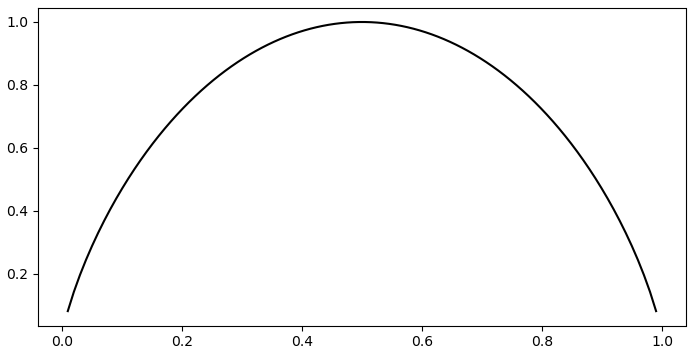2) 条件熵

3) 信息增益

$g\left(U|D\right)=H\left(U\right)-H\left(U|I\right)\phantom{\rule{0.278em}{0ex}}\phantom{\rule{0.278em}{0ex}}\phantom{\rule{0.278em}{0ex}}\phantom{\rule{0.278em}{0ex}}\phantom{\rule{0.278em}{0ex}}\phantom{\rule{0.278em}{0ex}}\phantom{\rule{0.278em}{0ex}}\left(8.4\right)$

### 2 决策树的生成之ID3与C4.5

#### 2.1 基本概念与定义

1) 信息熵

$X$$X$是一个取值有限的离散型随机变量（例如上一小节中可能夺冠的16只球队），其概率分布为$P\left(X={x}_{i}\right)={p}_{i},\phantom{\rule{0.278em}{0ex}}i=1,2,...,n$$P(X=x_i)=p_i,\;i=1,2,...,n$（每个球队可能夺冠的概率），则随机变量$X$$X$的信息熵定义为

$H\left(X\right)=-\sum _{i=1}^{n}{p}_{i}\mathrm{log}{p}_{i}\phantom{\rule{0.278em}{0ex}}\phantom{\rule{0.278em}{0ex}}\phantom{\rule{0.278em}{0ex}}\phantom{\rule{0.278em}{0ex}}\phantom{\rule{0.278em}{0ex}}\phantom{\rule{0.278em}{0ex}}\phantom{\rule{0.278em}{0ex}}\phantom{\rule{0.278em}{0ex}}\left(8.5\right)$

##### 2) 条件熵

$H\left(Y|X\right)=\sum _{i=1}^{n}{p}_{i}H\left(Y|X={x}_{i}\right)\phantom{\rule{0.278em}{0ex}}\phantom{\rule{0.278em}{0ex}}\phantom{\rule{0.278em}{0ex}}\phantom{\rule{0.278em}{0ex}}\phantom{\rule{0.278em}{0ex}}\phantom{\rule{0.278em}{0ex}}\phantom{\rule{0.278em}{0ex}}\left(8.6\right)$

3) 信息增益

$g\left(D,A\right)=H\left(D\right)-H\left(D|A\right)\phantom{\rule{0.278em}{0ex}}\phantom{\rule{0.278em}{0ex}}\phantom{\rule{0.278em}{0ex}}\phantom{\rule{0.278em}{0ex}}\phantom{\rule{0.278em}{0ex}}\phantom{\rule{0.278em}{0ex}}\phantom{\rule{0.278em}{0ex}}\left(8.7\right)$

• 数据集$D$$D$的经验熵$H\left(D\right)$$H(D)$

$H\left(D\right)=-\sum _{k=1}^{K}\frac{|{C}_{k}|}{|D|}{\mathrm{log}}_{2}\frac{|{C}_{k}|}{|D|}\phantom{\rule{0.278em}{0ex}}\phantom{\rule{0.278em}{0ex}}\phantom{\rule{0.278em}{0ex}}\phantom{\rule{0.278em}{0ex}}\phantom{\rule{0.278em}{0ex}}\phantom{\rule{0.278em}{0ex}}\phantom{\rule{0.278em}{0ex}}\left(8.8\right)$

从式$\left(8.8\right)$$(8.8)$可以看出，它计算的是“任意样本属于其中一个类别”这句话所包含的信息量。

• 数据集$D$$D$在特征值$A$$A$下的经验条件熵$H\left(D|A\right)$$H(D|A)$

$H\left(D|A\right)=\sum _{i=1}^{n}\frac{|{D}_{i}|}{|D|}H\left({D}_{i}\right)=-\sum _{i=1}^{n}\frac{|{D}_{i}|}{|D|}\sum _{k=1}^{K}\frac{|{D}_{ik}|}{|{D}_{i}|}{\mathrm{log}}_{2}\frac{|{D}_{ik}|}{|{D}_{i}|}\phantom{\rule{0.278em}{0ex}}\phantom{\rule{0.278em}{0ex}}\phantom{\rule{0.278em}{0ex}}\phantom{\rule{0.278em}{0ex}}\phantom{\rule{0.278em}{0ex}}\phantom{\rule{0.278em}{0ex}}\phantom{\rule{0.278em}{0ex}}\left(8.9\right)$

从式$\left(8.9\right)$$(8.9)$可以看出，它计算的是特征$A$$A$在各个取值条件下“任意样本属于其中一个类别”这句话所包含的信息量。

• 信息增益为

$g\left(D,A\right)=H\left(D\right)-H\left(D|A\right)\phantom{\rule{0.278em}{0ex}}\phantom{\rule{0.278em}{0ex}}\phantom{\rule{0.278em}{0ex}}\phantom{\rule{0.278em}{0ex}}\phantom{\rule{0.278em}{0ex}}\phantom{\rule{0.278em}{0ex}}\phantom{\rule{0.278em}{0ex}}\phantom{\rule{0.278em}{0ex}}\left(8.10\right)$

#### 2.2 计算示例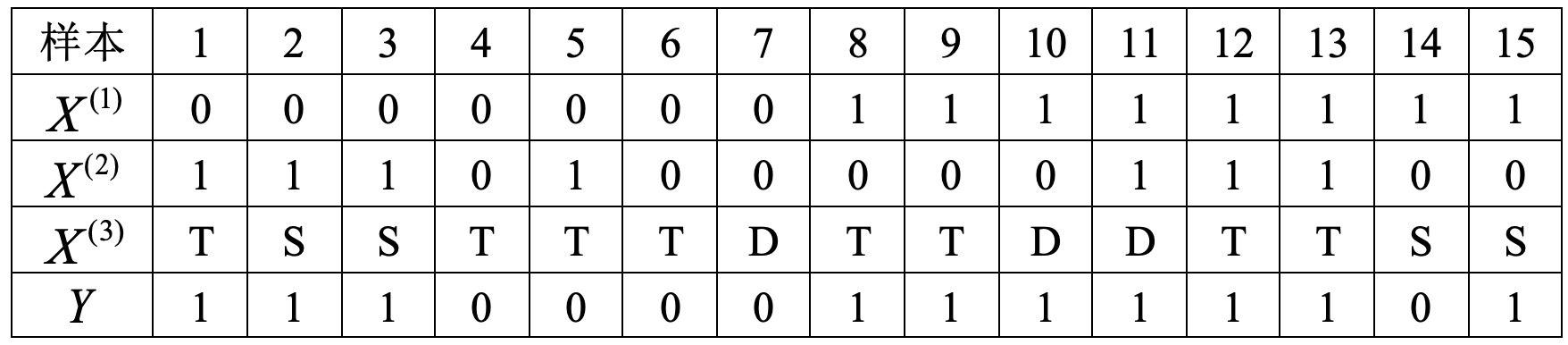1) 计算信息熵

$H\left(D\right)=-\left(\frac{5}{15}{\mathrm{log}}_{2}\frac{5}{15}+\frac{10}{15}{\mathrm{log}}_{2}\frac{10}{15}\right)\approx 0.918\phantom{\rule{0.278em}{0ex}}\phantom{\rule{0.278em}{0ex}}\phantom{\rule{0.278em}{0ex}}\phantom{\rule{0.278em}{0ex}}\phantom{\rule{0.278em}{0ex}}\phantom{\rule{0.278em}{0ex}}\phantom{\rule{0.278em}{0ex}}\left(8.11\right)$

2) 计算条件熵

• 已知外部信息“工作”的情况下有

$\begin{array}{rl}& H\left(D|{A}_{1}\right)=\left[\frac{7}{15}H\left({D}_{1}\right)+\frac{8}{15}H\left({D}_{2}\right)\right]\\ & =-\frac{7}{15}\left(\frac{3}{7}{\mathrm{log}}_{2}\frac{3}{7}+\frac{4}{7}{\mathrm{log}}_{2}\frac{4}{7}\right)-\frac{8}{15}\left(\frac{7}{8}{\mathrm{log}}_{2}\frac{7}{8}+\frac{1}{8}{\mathrm{log}}_{2}\frac{1}{8}\right)\approx 0.75\end{array}\phantom{\rule{0.278em}{0ex}}\phantom{\rule{0.278em}{0ex}}\phantom{\rule{0.278em}{0ex}}\phantom{\rule{0.278em}{0ex}}\phantom{\rule{0.278em}{0ex}}\phantom{\rule{0.278em}{0ex}}\phantom{\rule{0.278em}{0ex}}\left(8.12\right)$

$\left(8.12\right)$$(8.12)$中，${D}_{1},{D}_{2}$$D_1,D_2$分别是${A}_{1}$$A_1$取值为“无工作”和“有工作”时，训练样本划分后对应的子集。

• 已知外部信息“房子”的情况下有

$\begin{array}{rl}& H\left(D|{A}_{2}\right)=\left[\frac{8}{15}H\left({D}_{1}\right)+\frac{7}{15}H\left({D}_{2}\right)\right]\\ & =-\frac{8}{15}\left(\frac{4}{8}{\mathrm{log}}_{2}\frac{4}{8}+\frac{4}{8}{\mathrm{log}}_{2}\frac{4}{8}\right)-\frac{7}{15}\left(\frac{1}{7}{\mathrm{log}}_{2}\frac{1}{7}+\frac{6}{7}{\mathrm{log}}_{2}\frac{6}{7}\right)\approx 0.81\end{array}\phantom{\rule{0.278em}{0ex}}\phantom{\rule{0.278em}{0ex}}\phantom{\rule{0.278em}{0ex}}\phantom{\rule{0.278em}{0ex}}\phantom{\rule{0.278em}{0ex}}\phantom{\rule{0.278em}{0ex}}\phantom{\rule{0.278em}{0ex}}\left(8.13\right)$

$\left(8,13\right)$$(8,13)$中，${D}_{1},{D}_{2}$$D_1,D_2$分别是${A}_{2}$$A_2$取值为“无房”和“有房”时，训练样本划分后对应的子集。

• 已知外部信息“学历”的情况下有

$\begin{array}{rl}& H\left(D|{A}_{3}\right)=\left[\frac{3}{15}H\left({D}_{1}\right)+\frac{4}{15}H\left({D}_{2}\right)+\frac{8}{15}H\left({D}_{3}\right)\right]\\ & =-\frac{3}{15}\left(\frac{1}{3}{\mathrm{log}}_{2}\frac{1}{3}+\frac{2}{3}{\mathrm{log}}_{2}\frac{2}{3}\right)-\frac{4}{15}\left(\frac{1}{4}{\mathrm{log}}_{2}\frac{1}{4}+\frac{3}{4}{\mathrm{log}}_{2}\frac{3}{4}\right)\\ & -\frac{8}{15}\left(\frac{3}{8}{\mathrm{log}}_{2}\frac{3}{8}+\frac{5}{8}{\mathrm{log}}_{2}\frac{5}{8}\right)\approx 0.91\end{array}\phantom{\rule{0.278em}{0ex}}\phantom{\rule{0.278em}{0ex}}\phantom{\rule{0.278em}{0ex}}\phantom{\rule{0.278em}{0ex}}\phantom{\rule{0.278em}{0ex}}\phantom{\rule{0.278em}{0ex}}\phantom{\rule{0.278em}{0ex}}\left(8.14\right)$

$\left(8.14\right)$$(8.14)$中，${D}_{1},{D}_{2},{D}_{3}$${{D}_{1}},{{D}_{2}},{{D}_{3}}$分别是${A}_{3}$${{A}_{3}}$取值为“D”、“S”和“T”时，训练样本划分后对应的子集。

3) 计算信息增益

$\begin{array}{rl}& g\left(D,{A}_{1}\right)=0.918-0.75=0.168\\ & g\left(D,{A}_{2}\right)=0.918-0.81=0.108\\ & g\left(D,{A}_{3}\right)=0.918-0.91=0.08\end{array}\phantom{\rule{0.278em}{0ex}}\phantom{\rule{0.278em}{0ex}}\phantom{\rule{0.278em}{0ex}}\phantom{\rule{0.278em}{0ex}}\phantom{\rule{0.278em}{0ex}}\phantom{\rule{0.278em}{0ex}}\phantom{\rule{0.278em}{0ex}}\left(8.15\right)$

#### 2.3 ID3生成算法

ID3（Interactive Dichotomizer-3）算法的核心思想是在选择决策树的各个节点时，采用信息增益来作为特征选择的标准，从而递归地构建决策树。其过程可以概括为，从根节点开始计算所有可能划分情况下的信息增益；然后选择信息增益最大的特征作为划分特征，由该特征的不同取值建立子节点；最后对子节点递归地调用以上方法，构建决策树；直到所有特征的信息增益均很小或没有可以选择为止。例如根据8.2.2节最后的计算结果可知，首先应该将数据样本以特征${A}_{1}$$A_1$有无工作）作为划分方式对数据集进行第一次划分。下面开始介绍通过ID3来生成决策树的步骤及示例。

• 生成步骤

输入：训练数据集$D$$D$，特征集$A$$A$，阈值$\epsilon$$\varepsilon$;

输出：决策树。

(1) 若$D$$D$中所有样本属于同一类${C}_{k}$$C_k$（即此时只有一个类别）则T为单节点树，将${C}_{k}$$C_k$作为该节点的类标记，返回T;

(2) 若$A=\mathrm{\varnothing }$$A=\emptyset$，则T为单节点树，并将$D$$D$中样本数最多的类${C}_{k}$$C_k$作为该节点的类标记，并返回T；

(3) 否则，计算$A$$A$中各特征对$D$$D$的信息增益，选择信息增益最大的特征${A}_{g}$$A_g$

(4) 如果${A}_{g}$$A_g$的信息增益小于阈值$\epsilon$$\varepsilon$，则置T为单节点树，并将$D$$D$中样本数最多的类${C}_{k}$$C_k$作为该节点的类标记，返回T；

(5) 否则，对${A}_{g}$$A_g$的每一个可能值${a}_{i}$$a_i$，以${A}_{g}={a}_{i}$$A_g=a_i$$D$$D$分割为若干非空子集，并建立为子节点；

(6) 对于第$i$$i$个子节点，以${D}_{i}$$D_i$为训练集，以$A-\left\{{A}_{g}\right\}$$A-\{A_g\}$为特征集，递归地调用(1)-(5)，得到子树${T}_{i}$$T_i$，返回${T}_{i}$$T_i$

• 生成示例

下面就用ID3算法来对表8-1中的数据样本进行决策树生成示例。易知该数据集不满足步骤(1)(2)中的条件，所以开始执行步骤(3)。同时，根据8.2.2小节最后的计算结果可知，对于特征${A}_{1},{A}_{2},{A}_{3}$$A_1,A_2,A_3$来说，在${A}_{1}$$A_1$条件下信息增益最大，所以应该选择特征${A}_{1}$$A_1$作为决策树的根节点。

由于本例中未设置阈值，所以接着执行步骤(5)按照${A}_{1}$$A_1$的取值将训练集$D$$D$划分为两个子集${D}_{1},{D}_{2}$$D_1,D_2$，如表8-2所示。

表8-2 第一次划分表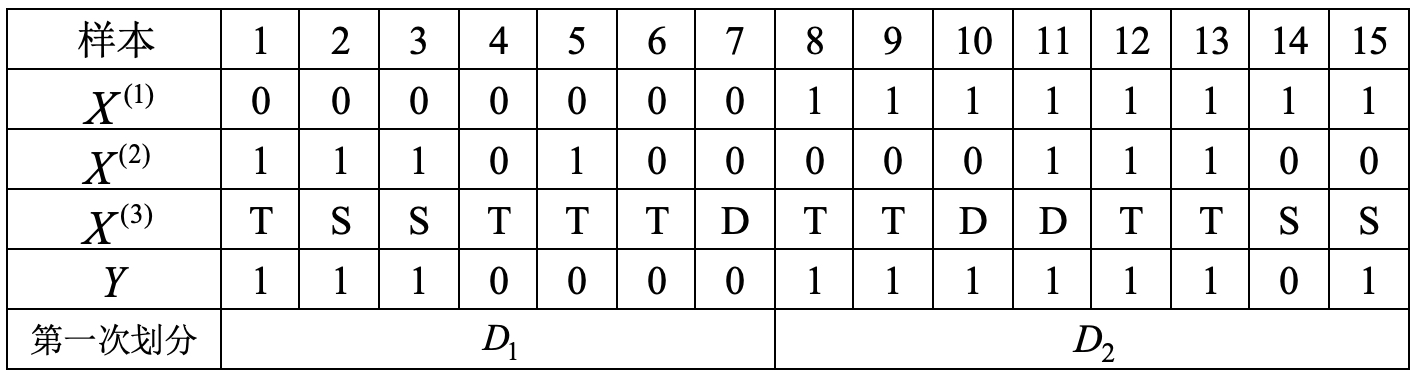接着开始执行步骤(6)，由于${D}_{1},{D}_{2}$$D_1,D_2$均不满足步骤(1)(2)中的条件，所以两部分需要分别继续执行后续步骤，此时生成的决策树如图8-3所示。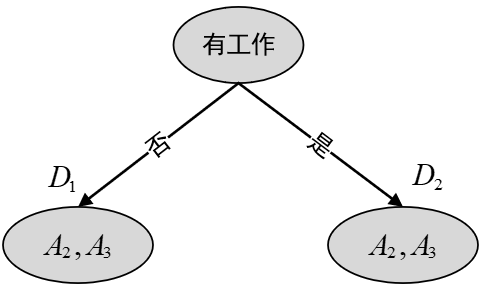图8-3 第一次划分

此时，对于子集${D}_{1}$$D_1$来说，需要从特征$A-\left\{{A}_{g}\right\}$$A-\{A_g\}$中即${A}_{2},{A}_{3}$$A_2,A_3$中选择新的特征，并计算信息增益。

1) 计算信息熵$H\left({D}_{1}\right)$$H(D_1)$

$H\left({D}_{1}\right)=-\left(\frac{3}{7}{\mathrm{log}}_{2}\frac{3}{7}+\frac{4}{7}{\mathrm{log}}_{2}\frac{4}{7}\right)\approx 0.985\phantom{\rule{0.278em}{0ex}}\phantom{\rule{0.278em}{0ex}}\phantom{\rule{0.278em}{0ex}}\phantom{\rule{0.278em}{0ex}}\phantom{\rule{0.278em}{0ex}}\phantom{\rule{0.278em}{0ex}}\phantom{\rule{0.278em}{0ex}}\left(8.16\right)$

2) 计算条件熵

$H\left({D}_{1}|{A}_{2}\right)=-\frac{3}{7}\left(\frac{3}{3}{\mathrm{log}}_{2}\frac{3}{3}+0\right)-\frac{4}{7}\left(\frac{1}{4}{\mathrm{log}}_{2}\frac{1}{4}+\frac{3}{4}{\mathrm{log}}_{2}\frac{3}{4}\right)\approx 0.464\phantom{\rule{0.278em}{0ex}}\phantom{\rule{0.278em}{0ex}}\phantom{\rule{0.278em}{0ex}}\phantom{\rule{0.278em}{0ex}}\phantom{\rule{0.278em}{0ex}}\phantom{\rule{0.278em}{0ex}}\phantom{\rule{0.278em}{0ex}}\left(8.17\right)$
$H\left({D}_{1}|{A}_{3}\right)=-\frac{1}{7}\left(0+0\right)-\frac{2}{7}\left(0+0\right)-\frac{4}{7}\left(\frac{3}{4}{\mathrm{log}}_{2}\frac{3}{4}+\frac{1}{4}{\mathrm{log}}_{2}\frac{1}{4}\right)\approx 0.464\phantom{\rule{0.278em}{0ex}}\phantom{\rule{0.278em}{0ex}}\phantom{\rule{0.278em}{0ex}}\phantom{\rule{0.278em}{0ex}}\phantom{\rule{0.278em}{0ex}}\phantom{\rule{0.278em}{0ex}}\phantom{\rule{0.278em}{0ex}}\left(8.18\right)$

根据式$\left(8.17\right)$$(8.17)$$\left(8.18\right)$$(8.18)$的结果可知，对于子集${D}_{1}$$D_1$来说，无论其采用${A}_{2},{A}_{3}$$A_2,A_3$中的哪一个特征进行划分，最后计算得到的信息增益都是相等的，所以这里不妨就以特征${A}_{2}$$A_2$进行划分。

同理，对于子集${D}_{2}$${{D}_{2}}$来说，也需要从特征$A-\left\{{A}_{g}\right\}$$A-\{A_g\}$中即${A}_{2},{A}_{3}$$A_2,A_3$中选择新的特征，并计算信息增益。

1) 计算信息熵

$H\left({D}_{2}\right)=-\left(\frac{1}{8}{\mathrm{log}}_{2}\frac{1}{8}+\frac{7}{8}{\mathrm{log}}_{2}\frac{7}{8}\right)\approx 0.544\phantom{\rule{0.278em}{0ex}}\phantom{\rule{0.278em}{0ex}}\phantom{\rule{0.278em}{0ex}}\phantom{\rule{0.278em}{0ex}}\phantom{\rule{0.278em}{0ex}}\phantom{\rule{0.278em}{0ex}}\phantom{\rule{0.278em}{0ex}}\left(8.19\right)$

2) 计算条件熵

$H\left({D}_{2}|{A}_{2}\right)=-\frac{5}{8}\left(\frac{1}{5}{\mathrm{log}}_{2}\frac{1}{5}+\frac{4}{5}{\mathrm{log}}_{2}\frac{4}{5}\right)-\frac{3}{8}\left(0+0\right)\approx 0.451\phantom{\rule{0.278em}{0ex}}\phantom{\rule{0.278em}{0ex}}\phantom{\rule{0.278em}{0ex}}\phantom{\rule{0.278em}{0ex}}\phantom{\rule{0.278em}{0ex}}\phantom{\rule{0.278em}{0ex}}\phantom{\rule{0.278em}{0ex}}\left(8.20\right)$
$H\left({D}_{2}|{A}_{3}\right)=-\frac{2}{8}\left(0+0\right)-\frac{2}{8}\left(\frac{1}{2}{\mathrm{log}}_{2}\frac{1}{2}+\frac{1}{2}{\mathrm{log}}_{2}\frac{1}{2}\right)-\frac{4}{8}\left(0+0\right)=0.25\phantom{\rule{0.278em}{0ex}}\phantom{\rule{0.278em}{0ex}}\phantom{\rule{0.278em}{0ex}}\phantom{\rule{0.278em}{0ex}}\phantom{\rule{0.278em}{0ex}}\phantom{\rule{0.278em}{0ex}}\phantom{\rule{0.278em}{0ex}}\left(8.21\right)$

3) 信息增益

$\begin{array}{rl}& g\left({D}_{2}|{A}_{2}\right)=0.544-0.451=0.093\\ & g\left({D}_{2}|{A}_{3}\right)=0.544-0.25=0.294\end{array}\phantom{\rule{0.278em}{0ex}}\phantom{\rule{0.278em}{0ex}}\phantom{\rule{0.278em}{0ex}}\phantom{\rule{0.278em}{0ex}}\phantom{\rule{0.278em}{0ex}}\phantom{\rule{0.278em}{0ex}}\phantom{\rule{0.278em}{0ex}}\left(8.22\right)$

根据式$\left(8.22\right)$$(8.22)$的计算结果可知，对于子集${D}_{2}$$D_2$来说，采用特征${A}_{3}$$A_3$来对其进行划分时所产生的信息增益最大，因此应该选择特征${A}_{3}$$A_3$来作为子集${D}_{2}$${{D}_{2}}$的根节点。

到此，根据上述计算过程，便可以得到第二次划分后的结果，如表8-3所示。

表8-3 第二次划分表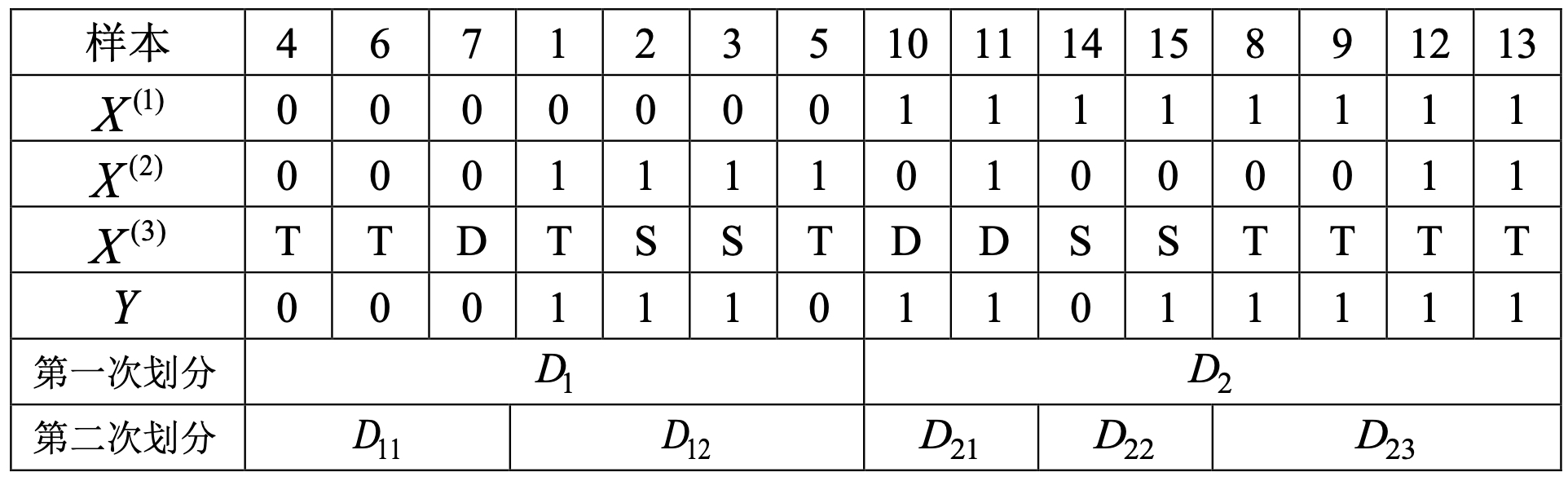从表8-3中的结果可知，${D}_{11},{D}_{21},{D}_{23}$$D_{11},D_{21},D_{23}$这三个子集中样本均只有一个类别，既满足生成步骤中的第(1)步，故此时可以得到第二次划分后的决策树，如图8-4所示。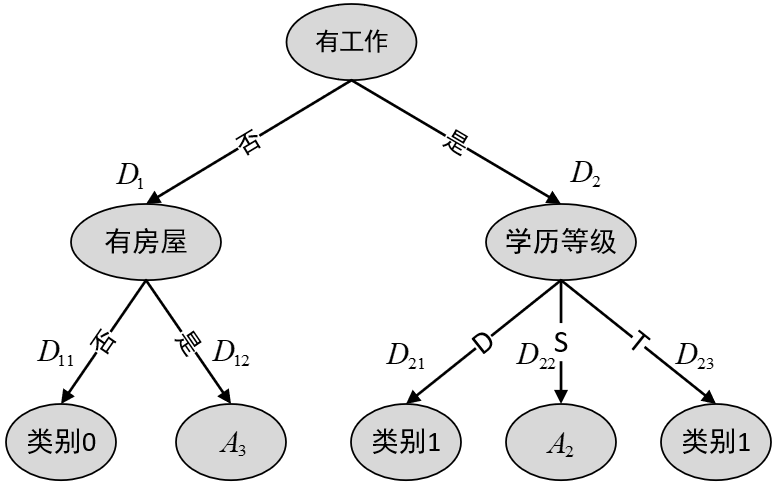图8-4 第二次划分

由于子集${D}_{12},{D}_{22}$$D_{12},D_{22}$均不满足终止条件(2)(4)，且此时两个子集中均只有一个特征可以选择，所以并不需要再进行比较直接划分即可得，如表8-4所示。

表8-4 第三次划分表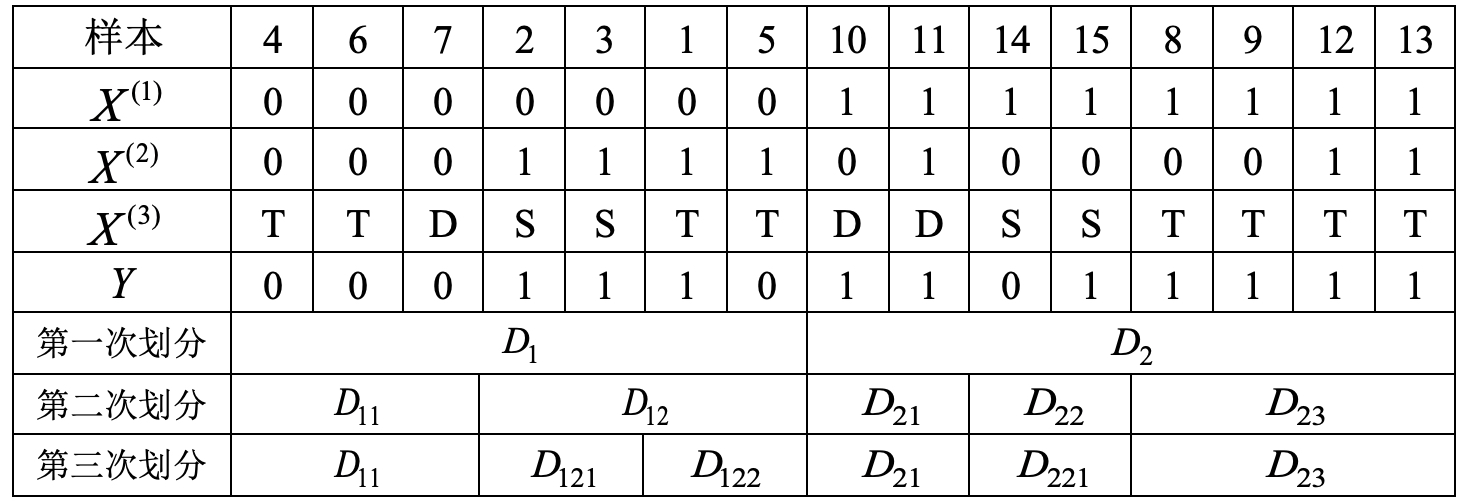根据表8-4中的结果可知，子集${D}_{121}$$D_{121}$满足生成步骤中的第(1)步，故此时可以得到第三次划分后的决策树，如图8-5所示。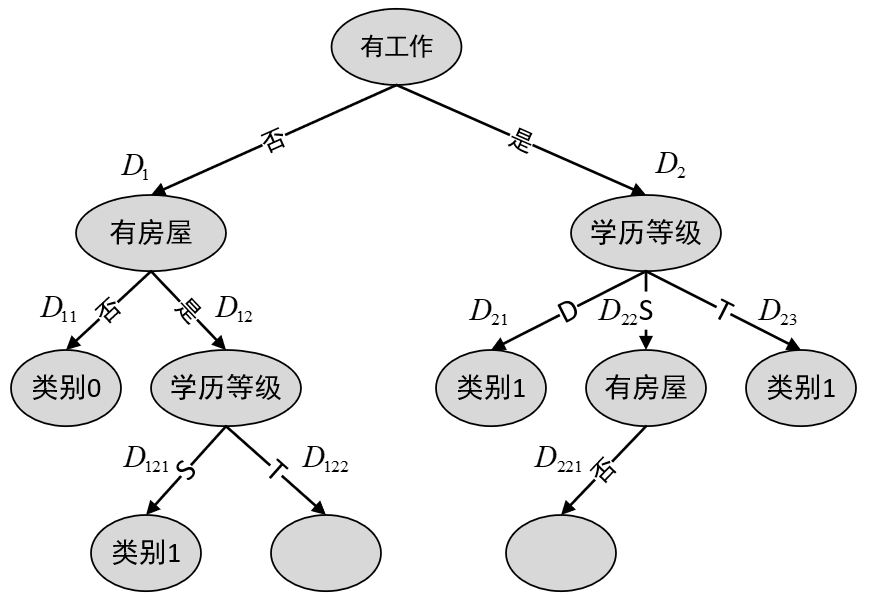图8-5 第三次划分

此时，由于子集${D}_{122},{D}_{221}$$D_{122},D_{221}$满足生成步骤中第 (2)步的终止条件，即再无特征可以进行划分，需要选择样本数最多的类别作为该节点的类别进行返回。但巧合的是子集${D}_{122},{D}_{221}$$D_{122},D_{221}$中不同类别均只有一个样本，因此随机选择一个类别即可。不过在实际过程中很少会出现这样的情况，因为一般当节点的样本数小于某个阈值时也会停止继续划分。这样便能得到最终生成的决策树，如图8-6所示。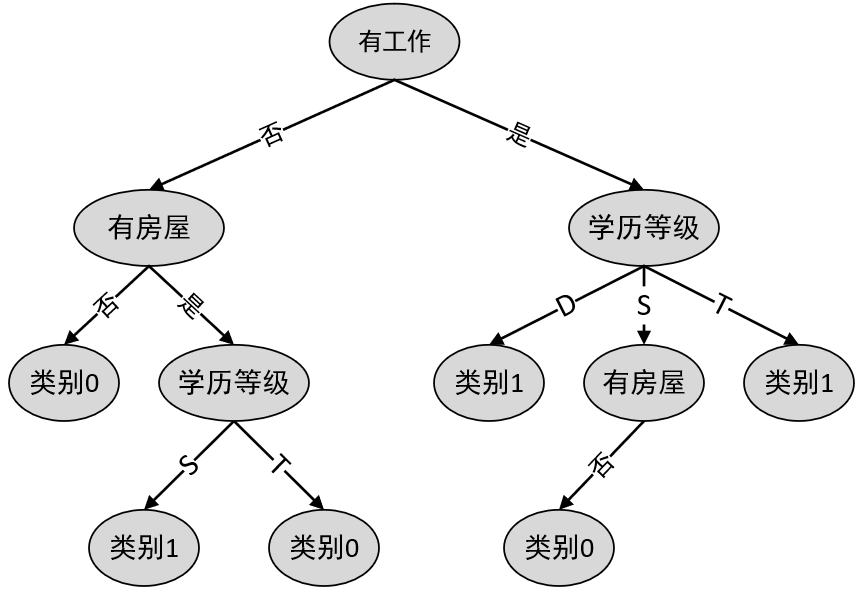#### 2.4 C4.5生成算法

${g}_{R}\left(D,A\right)=\frac{g\left(D,A\right)}{{H}_{A}\left(D\right)}\phantom{\rule{0.278em}{0ex}}\phantom{\rule{0.278em}{0ex}}\phantom{\rule{0.278em}{0ex}}\phantom{\rule{0.278em}{0ex}}\phantom{\rule{0.278em}{0ex}}\phantom{\rule{0.278em}{0ex}}\phantom{\rule{0.278em}{0ex}}\left(8.23\right)$

1. 计算得到信息增益
$\begin{array}{rl}& g\left(D,{A}_{1}\right)=0.918-0.75=0.168\\ & g\left(D,{A}_{2}\right)=0.918-0.81=0.108\\ & g\left(D,{A}_{3}\right)=0.918-0.91=0.08\end{array}\phantom{\rule{0.278em}{0ex}}\phantom{\rule{0.278em}{0ex}}\phantom{\rule{0.278em}{0ex}}\phantom{\rule{0.278em}{0ex}}\phantom{\rule{0.278em}{0ex}}\phantom{\rule{0.278em}{0ex}}\phantom{\rule{0.278em}{0ex}}\left(8.24\right)$
1. 计算各特征的信息熵